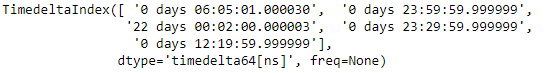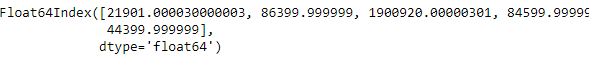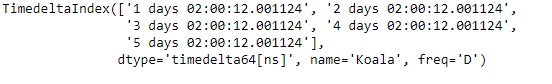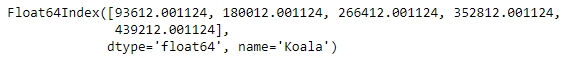Related Articles
Python | Pandas TimedeltaIndex.total_seconds()
• Last Updated : 06 Jan, 2019

Python is a great language for doing data analysis, primarily because of the fantastic ecosystem of data-centric python packages. Pandas is one of those packages and makes importing and analyzing data much easier.

Pandas` TimedeltaIndex.total_seconds()` function is used to find the total duration of each element expressed in seconds. It returns an index object.

Syntax : TimedeltaIndex.total_seconds()

Parameters : None

Return : index

Example #1: Use `TimedeltaIndex.total_seconds()` function to find out the total time duration of each element of the given TimedeltaIndex object expressed in seconds.

 `# importing pandas as pd``import` `pandas as pd`` ` `# Create the TimedeltaIndex object``tidx ``=` `pd.TimedeltaIndex(data ``=``[``'06:05:01.000030'``, ``'+23:59:59.999999'``,``                        ``'22 day 2 min 3us 10ns'``, ``'+23:29:59.999999'``, ``                        ``'+12:19:59.999999'``])`` ` `# Print the TimedeltaIndex object``print``(tidx)`

Output :Now we will use the `TimedeltaIndex.total_seconds()` function to find the total seconds for each element of the tidx object.

 `# time duration of each element expressed in seconds``tidx.total_seconds()`

Output :As we can see in the output, the `TimedeltaIndex.total_seconds()` function has returned an index object containing the time duration for each element of the TimedeltaIndex object expressed in seconds.

Example #2: Use `TimedeltaIndex.total_seconds()` function to find out the total time duration of each element of the given TimedeltaIndex object expressed in seconds.

 `# importing pandas as pd``import` `pandas as pd`` ` `# Create the TimedeltaIndex object``tidx ``=` `pd.TimedeltaIndex(start ``=``'1 days 02:00:12.001124'``,``                        ``periods ``=` `5``, freq ``=``'D'``, name ``=``'Koala'``)`` ` `# Print the TimedeltaIndex object``print``(tidx)`

Output :Now we will use the `TimedeltaIndex.total_seconds()` function to find the total seconds for each element of the tidx object.

 `# time duration of each element expressed in seconds``tidx.total_seconds()`

Output :As we can see in the output, the `TimedeltaIndex.total_seconds()` function has returned an index object containing the time duration for each element of the TimedeltaIndex object expressed in seconds.

Attention geek! Strengthen your foundations with the Python Programming Foundation Course and learn the basics.

To begin with, your interview preparations Enhance your Data Structures concepts with the Python DS Course.

My Personal Notes arrow_drop_up